How Cheenta works to ensure student success?
Explore the Back-Story

# Sequence and Series: IIT JAM 2016 Problem 24## Sequence and series of real numbers

A sequence of real numbers is an one to one mapping from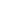(the set of natural numbers) to a subset of(the set of all real numbers).

Sum of the terms of a real sequence sequence is called a series.

## Try the problem

Find the sum of the series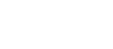.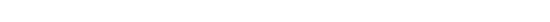IIT JAM 2016, PROBLEM 24

Sequence and Series of real numbers.

6 out of 10

Introduction to Real Analysis : Robert G. Bartle & Donald R. Sherbert.

## Use some hints

This problem is a very basic one, this problem can easily be solved by step by step solution. The steps are:

Step 1 : First we will ignore the summation part. We will factorize the denominator, because we are going step by step so our aim is to simplify the given problem first.

Step 2: After factorizing the the denominator we will reach to a position where we have to use partial fraction to go forward.

Step 3: In this step we will take care of the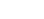part, like how it will affect the series.

Step 4: After taking care of thewe will now expand the summation (breaking it into infinite sum).

Step 5 : So after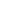steps we are halfway done now just the last simplification is left we will use the value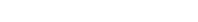to simplify it further.

Now try to solve the entire problem by following these steps !!!

Let us see how to execute STEP 1 ., here we are only concerned with the denominator part so,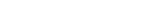So we have successfully factorized the denominator. Now you can reach to the part where we have to use the concept of partial fraction!!!

Let us execute STEP 2 now.

After factorizing the denominator in HINT 1 we get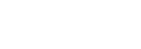Now our aim here is to seperate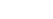andso we can do this by using partial fraction.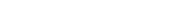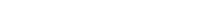Now taking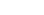we get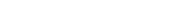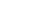Again taking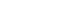, we get: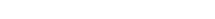therefore,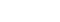So we get,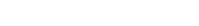So our aim here is successful we have separatedand. Now can you proceed further ???

Now after using partial fraction we get.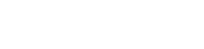Now we will execute STEP 4 i.e., we will take care of theand at the same time we will execute STEP 4 i.e, we will split it into the infinite sum.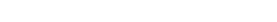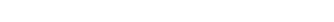Now after this can you applying the formula of '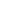' to finish the problem!!

Now only STEP 5 is left to execute. So we will use the infinite series of.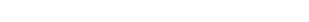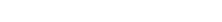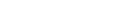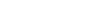Hence the anser is C.

## Sequence and series of real numbers

A sequence of real numbers is an one to one mapping from(the set of natural numbers) to a subset of(the set of all real numbers).

Sum of the terms of a real sequence sequence is called a series.

## Try the problem

Find the sum of the series.IIT JAM 2016, PROBLEM 24

Sequence and Series of real numbers.

6 out of 10

Introduction to Real Analysis : Robert G. Bartle & Donald R. Sherbert.

## Use some hints

This problem is a very basic one, this problem can easily be solved by step by step solution. The steps are:

Step 1 : First we will ignore the summation part. We will factorize the denominator, because we are going step by step so our aim is to simplify the given problem first.

Step 2: After factorizing the the denominator we will reach to a position where we have to use partial fraction to go forward.

Step 3: In this step we will take care of thepart, like how it will affect the series.

Step 4: After taking care of thewe will now expand the summation (breaking it into infinite sum).

Step 5 : So aftersteps we are halfway done now just the last simplification is left we will use the valueto simplify it further.

Now try to solve the entire problem by following these steps !!!

Let us see how to execute STEP 1 ., here we are only concerned with the denominator part so,So we have successfully factorized the denominator. Now you can reach to the part where we have to use the concept of partial fraction!!!

Let us execute STEP 2 now.

After factorizing the denominator in HINT 1 we getNow our aim here is to seperateandso we can do this by using partial fraction.Now takingwe getAgain taking, we get:therefore,So we get,So our aim here is successful we have separatedand. Now can you proceed further ???

Now after using partial fraction we get.Now we will execute STEP 4 i.e., we will take care of theand at the same time we will execute STEP 4 i.e, we will split it into the infinite sum.Now after this can you applying the formula of '' to finish the problem!!

Now only STEP 5 is left to execute. So we will use the infinite series of.Hence the anser is C.

## Subscribe to Cheenta at Youtube

This site uses Akismet to reduce spam. Learn how your comment data is processed.

### Knowledge Partner